S k i l l
i n
A R I T H M E T I C

Lesson 20

# UNIT FRACTIONS

In this Lesson, we will answer the following:

1. What is a unit fraction?
2. How can we express a whole number as a fraction?
3. What do we mean by the complement of a proper fraction?

Section 2

4. What kind of fraction does "out of" indicate?

 1. What is a unit fraction? A fraction whose numerator is 1.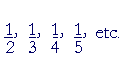A unit, recall, is whatever we call one. (Lesson 1.)  Each unit fraction is a part of number 1.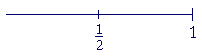12 is one half of 1.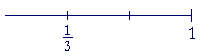13 is the third part of 1.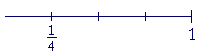14 is the fourth part of 1.

And so on.

 Example 1.    In the fraction 45 , what number is the unit, and how many

of them are there?

Answer.  The denominator of a fraction names the unit -- the part of 1.  The numerator tells their number -- how many.

 In the fraction 45 , the unit is 15 .  And there are 4 of them.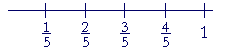Example 2.   Let 13 be the unit, and count to 2 13 .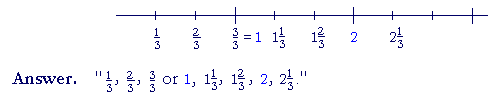We see that every fraction is a multiple of some unit fraction:

 23 =  2 × 13 = 13 + 13 .
 35 =  3 × 15 = 15 + 15 + 15 .
 Example 3.   Add 28 + 38 .
 Answer. 58 .
 2 eighths + 3 eighths are 5 eighths. The unit is 18 .

This illustrates the following principle:

In addition and subtraction, the units must be the same.

We will see this in Lesson 24.  In any fraction, the denominator names the unit.

 79 − 39 = 49

Example 4.   1 is how many fifths?

 Answer. 55 .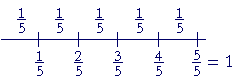15 is contained in 1 five times.

Similarly,

 1 = 33 = 44 = 1010

And so on.  We may write 1 with any denominator.  Which is to say, we may decompose 1 into any parts:  Halves, thirds, fourths, fifths, millionths.

 Example 5.   Add, and express the sum as an improper fraction: 59 + 1.
 Answer. 59 + 1 = 59 + 99 = 14 9 .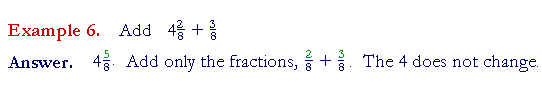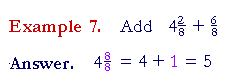2. How can we express a whole number as fraction?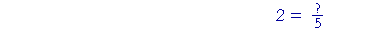Multiply the whole number by the denominator. That product will be the numerator.

 Example 8.    2 = 2 × 5   5 = 10 5 .
 Since 1 = 55 , then 2 is twice as many fifths:  2 = 10 5 .
 Example 9.    6 = ?3
 Answer.    6  = 6 × 3   3 = 18 3 .
 Example 10.     How many times is 18 contained in 5?  That is, 5 = ?8 .
 Answer.   5  = 40 8 .

The complement of a proper fraction

 3. What do we mean by the complement of a proper fraction?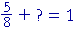It is the proper fraction we must add in order to get 1.

 Example 11. 58 + ? = 1
 Answer.   Since 1 = 88 , then 58 + 38 = 1

Equivalently, since finding what number to add  is subtraction,

 1 − 58 = 38 .
 38 is called the complement of 58 . 38 completes 58 to make 1.
 Example 12.    How much is 1 − 13 ?
 Answer.   1 − 13 = 23 .
 13 plus 23 = 33 , which is 1.
 Example 13.    1 − 25 = 35 .
 When we add 35 to 25 ,  we get 1.

 Example 14.   How much is 6 34 − 14 ?
 Answer.  6 24 .  The 6 is not affected.
 Example 15.   How much is 6 44 − 14 ?
 Answer.  6 34 .
 But 6 44 is 7.  That is,
 7 − 14 =  6 34

Look at the fact: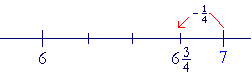We are subtracting 14 -- which is less than 1 -- from 7.  The answer
 therefore falls beween 6 and 7.  And 34 is the complement of 14 .

Compare

 1 − 14 :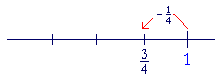In other words:

Whenever we subtract a proper fraction from a whole number greater than 1, the answer will be a mixed number which is one whole number less, and whose fraction is the complement of the proper fraction.

 Example 16. 5 − 13 = 4 23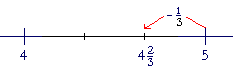4 is one less than 5.  And 23 is the complement of 13 .

On the other hand, we could say that we can only subtract thirds from thirds. Therefore we must create thirds by breaking off 1 from 5

 and calling it 33 .
 5 − 13 = 4 33 − 13 = 4 23 .
 Example 17.   9 − 25 =  8 35 .

We could check this by adding:

 8 35 + 25 = 9.

At this point, please "turn" the page and do some Problems.

or

Continue on to the next Section.

www.proyectosalonhogar.com